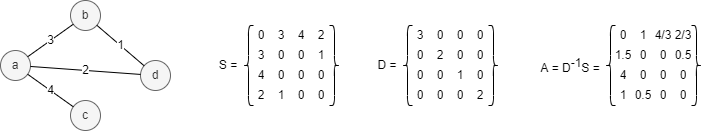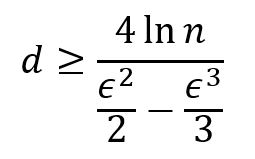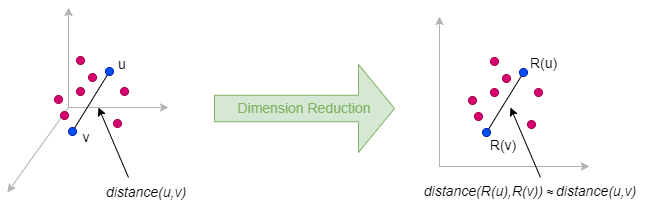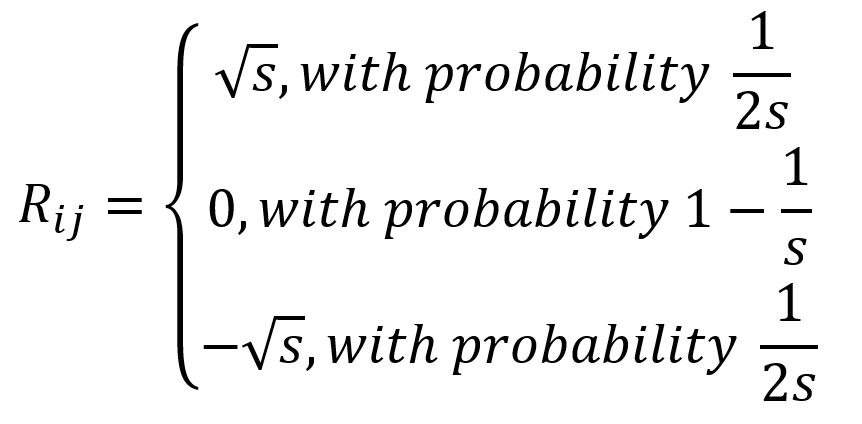# Change Nickname

Current Nickname:

Certifications

Certificate Issued at Valid until Serial No. File
Serial No. Valid until

Not having one? Apply now! >>>

Invoice

ProductName CreateTime ID Price File
ProductName CreateTime ID Price File

# Fast Random Projection

## Overview

Fast Random Projection, or FastRP in short, is a graph embedding algorithm that is observably faster than the traditional methods such as random walk. It allows the iterative computation of node embeddings so that the similarity matrix need not be explicitly constructed, which speeds up the algorithm. FastRP was proposed by H. Chen et al. in 2019.

Related materials of the algorithm:

## FastRP Framework

FastRP algorithm is a procedure with two components: first, similarity matrix construction, then dimension reduction by random projection to get the embeddinng result.

### Similarity Matrix Construction

FastRP keeps two key features when constructing node similarity matrix:

1. Preserve high-order proximity in the graph
2. Perform element-wise normalization on the matrix

Let S be the adjacency matrix of graph G =(V,E), D be the degree matrix, and A be the transition matrix and A = D-1S. See the example below, the number on each edge represents the edge weight:However, directly applying dimension reduction techniques on S or A is problematic. Real-world graphs are usually extremely sparse, which means most of the entries in S or A are 0. However, the absence of edge between two nodes does not imply that there is no association between them.

The fact is that in matrix A, entry Aij stands for the probablity of node i reaching to node j with 1-step random walk. Furthermore, if raises A to k-th power to get matrix Ak, then Akij denotes the probability of reaching node j from node i in k steps of random walk. Hence, matrix Ak perserves high-order proximity of the graph.

Now consider the normalization on matrix Ak. First, many Ak of the real-world has a heavy-tailed distribution, normalization is important to balance the influene of node degrees. In addition, it can be proved that Akij → dj/2m when k → ∞, where m = |E| is the number of edges in the graph, and dj is the degree of the j-th node.

Let's construct a normalization diagonal matrix L and entry Lij = (dj/2m)β, where β is the normalization strength. Normalization strength controls the impact of node degree on the embeddings: when β is negative, the importance of high-degree neighbors is weakened, and the opposite when β is positive.

### Matrix Dimensionality Deduction

#### Johnson–Lindenstrauss Lemma

FastRP is theoretically backed by Johnson–Lindenstrauss Lemma (or JL Lemma for short) originally proposed by W. Johnson and J. Lindenstrauss in 1984, after continued research by later generations, it is now generally accepted as:

A node set `M` in a high-dimensional Euclidean space can be embeded into a low-dimensional space via a linear projection `R` and approximately preserve pairwise distances among nodes, i.e.:where 0 < `ϵ` < 1 is an acceptable distance error, the embedding dimension `d` is irrelevant to the dimension of the original node set, but depends on the size of the node set `n = |M|` and `ϵ`:#### Random Projection

Random Projection is a simple but efficient approach of dimensionality reduction:

1. A `n×m` dataset `M` as input, where `n` is the number of elements, `m` is the original dimensionality (number of features)
2. Construct a random projection matrix `R` of `m×d`, where `d` is the dimensionality that intend to reduce to (`d ≪ n`)
3. Get the low-dimensional matrix `N` by simply calculating `N = M × R`, and the final dimensionality would be `n×d`.

Dimensionality `d` depends on the dataset's size `n`, not on the original dimensionality `m`. In FastRP algorithm, for graph data, we have `m = n`.

The difference among different random projection algorithms ismostly in the construction of `R`. FastRP adopts Very Sparse Random Projection, proposed by P. Li et al.:where `s=sqrt(m)`.

## Algorithm Process

The process of FastRP algorithm are as followed:

1. Generate the random projection matrix R
2. First iteration：generate dimensionality reduction matrix N1 = A ⋅ L ⋅ R
2. Second iteration: generate dimensionality reduction matrix N2 = A2 ⋅ L ⋅ R = A ⋅ N1
3. Iterate for k times: generate dimensionality reduction matrix Nk = A ⋅ Nk-1
4. Generate the final embedding results, i.e., N = α1N1 + ... + αkNk, where α1,...,αk are the iteration weights.

## Command and Configuration

• Command:`algo(fastRP)`
• Configurations for the parameter `params()`:
Name Type
Default
Specification
Description
dimension int / >=1 Dimension of node embedding vector
normalizationStrength float / / Normalization strength `β`
iterationWeights []float [0.0,1.0,1.0] >0 Weight for each iteration, the size of the array is the number of iterations
edge_schema_property []`@<schema>?.<property>` / Numeric edge property, LTE needed Edge weight property/properties, schema can be either carried or not; edge without any specified property does not participate in the calculation
node_schema_property []`@<schema>?.<property>` / Numeric node property, LTE needed Node property/properties to be used as features, schema can be either carried or not; node without any specified property does not participate in the calculation
propertyDimension int / (0,`dimension`] Random projection matrix `R` is formed by concatenation of two parts: the first part is formed by the Very Sparse Random Projection algorithm, the second part of length `propertyDimension` is a linear combination of the property vectors, using the property values of the node as weights
limit int -1 -1 or >=0 Number of results to return; return all results if sets to -1 or not set

## Algorithm Execution

#### 1. File Writeback

Configuration
Data in Each Column
filename `_id`, `embedding_result`

#### 2. Property Writeback

Not supported by this algorithm.

#### 3. Statistics Writeback

This algorithm has no statistics.

### Direct Return

Alias Ordinal Type
Description
Column Name
0 []perNode Node and its graph embedding results `_uuid`, `embedding_result`

### Streaming Return

Alias Ordinal Type
Description
Column Name
0 []perNode Node and its graph embedding results `_uuid`, `embedding_result`

### Real-time Statistics

This algorithm has no statistics.